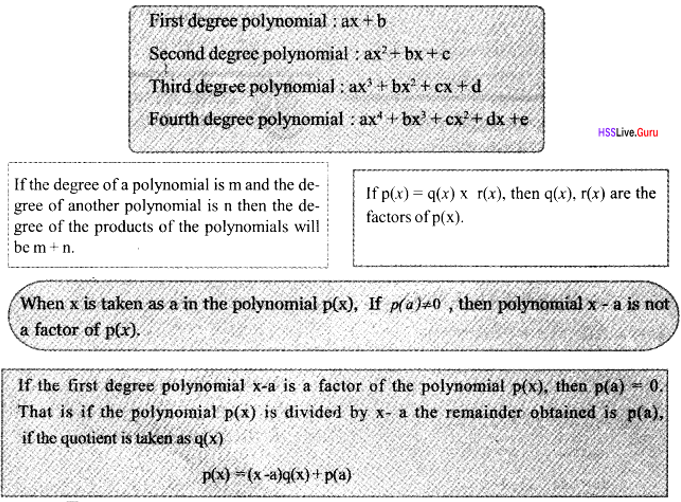# Kerala Syllabus 10th Standard Maths Solutions Chapter 10 Polynomials

## Kerala State Syllabus 10th Standard Maths Solutions Chapter 10 Polynomials

### Polynomials Textbook Questions & Answers

Textbook Page No. 237

Kerala Syllabus X Questions 1. Write the second degree polynomials. given below as the product of two first degree polynomials. Find also the solutions of the equation p(x) = 0 in each.
i. p(x) = x2 – 7x+12
ii. p(x) = x2 + 7x + 12
iii.p (x) = x2 – 8x +12
iv. p(x) = x2 + 13x +12
v. p (x) = x2 + 12x – 13
vi. p (x) = x2 – 12x – 13
i. p (x) = x2 – 7x + 12
a + b = –7, ab = 12
a = –3, b = –4
x2 – 7x + 12 = (x – 3) (x – 4)
x2 – 7x + 12 = 0
(x – 3) (x – 4) = 0
x – 3 = 0, x – 4 = 0
x = 3, x = 4
ii. p(x) = x2 + 7x + 12
a + b = 7, ab= 12 a = 3, b = 4
x2 + 7x + 12 = (x + 3) (x + 4)
x2 + 7x + 12 = 0
(x + 3)(x + 4) = 0
x = 3, x = 4
iii. p(x) = x2 – 8x + 12
a + b = 8, ab= 12
a = 6, b = –2
x2 – 8x + 12 = (x – 6) (x – 2)
(x – 6) (x – 2) = 0
x = 6, x = 2
iv. p(x) =x2 + 13x + 12
a+b = 13, ab = 12
a =12, b=1
(x2 +13x + 12) = (x + 12) (x + 1)
x2+13x + 12 = 0
(x+ 12) (x+ 1) = 0
x+ 12 = 0, x+ 1 = 0
x = -12 ,x = –1
v. p(x) = x2 + 12x – 13
a = –13, b = 1
x2 + 12x – 13 = (x + 13)(x – 1)
x + 13 = 0, x – 1 =0
x = –13, x= 1 .
vi. p(x) = x2 – 12x – 13
x2 – 12x – 13 = (x – a) (x – b)
= x2 – (a + b) x + ab
a + b= 12 ab = –13
(a – b)2 =(a + b)2 – 4ab
= (12)2 – 4x – 13 = 196
a – b = 14
a + b= 12
a= 13; b = –1
x2 – 12x – 13 = (x – 13)(x + 1)
x – 13 = 0, x + 1 =0
x= 13 ,x = –1Textbook Page No. 240

Polynomials Class 10 SCERT Questions 1. In each pair of polynomials given below, find the number to be subtracted from the first to get a polynomial for which the second is as factor. Find also the second factor of the polynomial got on subtracting the number.
i. x2 – 3x + 5, x – 4
ii. x2 – 3x + 5, x + 4
iii. x2 + 5x – 7, x – 1
iv. x2 – 4x – 3, x – 1
p(x) = x2 – 3x + 5
If x – 4 is a factor, p(4) = 0
p(4) = (4)2 – 3 x 4 + 5 = 9
For x – 4 to become a factor of p (4) must be equal to zero.
For p (4) = 0 here we have to subtract 9 from p(x).
That is, add -9 to p(x) for (x – 4) become a factor.
∴ p(x) = x2 – 3x + 5 – 9 = x2 – 3x – 4
x2 – 3x – 4 = (x – a) (x – b)
= x2 – (a + b) x + ab
a + b = 3
ab = –4
(a – b)2 = (a + b)2 – 4 ab
= (3)2 – 4x – 4 = 25
a – b = 5
a + b = 3
a = 4; b = –1
x2 – 3x – 4 = (x – 4)(x + 1)
Second factor is (x +1)
ii. p(x) = x2 – 3x + 5
If x + 4 is a factor, p(–4) = 0
p(–4) = (–4)2 – 3x – 4 + 5 = 33
For x + 4 to become a factor of p (–4)
must be equal to zero.
For p (–4) = 0 here we have to subtract 33 from p(x).
That is, add –33 to p(x) for (x + 4) become a factor.
∴p(x) = x2 – 3x + 5 – 33 = x2 – 3x – 28
x2 – 3x – 28 = (x – a) (x – b)
= x2 – (a+b) x + ab
a + b = 3
ab = -28
(a – b)2 = (a + b)2 – 4ab
= (3)2 – 4x – 28 = 121
a – b= 11
a + b = 3
a = 7;
b = -4 x2 – 3x – 28 = (x – 7)(x + 4)
Second factor is (x – 7)
iii. p(x) = x2 + 5x – 7
If x – 1 is a factor, p(1) = 0
p(1) = (1)2 +5 x 1 – 7 = –1
For x – 1 to become a factor of p (1) must be equal to zero.
For p (1) = 0 here we have to subtract –1 from p(x).
That is, add 1 to p(x) for (x – 1) become a factor.
p(x) = x2 + 5x – 7 + 1 = x2 + 5x – 6
x2 + 5x – 6 = (x – a) (x – b)
a + b = 5
ab = –6
a = –6; b= 1
x2 + 5x – 6 = (x + 6)(x – 1)
Second factor is (x + 6)
iv. p(x) = x2 – 4x – 3
If x – 1 is a factor, p(1) = 0
p(1) = (1)2 – 4 x 1 – 3 = –6
For x – 1 to become a factor of p (1) must
be equal to zero.
For p (1) = 0 here we have to subtract -6 from p(x).
That is, add 6 to p(x) for (x – 1) become a factor.
∴ p(x) = x2 – 4 x – 3 + 6 = x2 – 4x +3
x2 – 4x + 3 = (x – a) (x – b)
a + b = –4 ab = 3
a =1; b = 3
x2 – 4x +3 = (x – 1)(x – 3)
Second factor is (x – 3)

SSLC Maths Polynomials Questions 2. In the polynomial x2 + kx + 6, what number must be taken ask to get a polynomial for which x –1 is a factor? Find also the other factor of that polynomial.
p (x) = x2 + kx + 6
If (x – 1) is a factor of p(x)
then p(1) = 0
p(1) = 12 + k x 1 + 6 = 7 + k
7 + k = 0
k= –7
∴ p(x) = x2 – 7x + 6
a + b = 7
ab = 6
a= 1, b = 6
factors are (x – 1 )(x – 6)
Second factor is (x – 6)

Polynomials Class 10 Kerala Syllabus Questions 3. In the polynomial kx2 + 2x – 5, what number must be taken ask to get a polynomial for which x –1 is a factor?
p(x) = kx2 + 2x – 5
If (x – 1) is a factor, then p(1) = 0
p(1) = k(l)2 + 2 x 1 – 5
= k + 2 – 5 = k – 3
k – 3 = 0 k = 3

Textbook Page No. 242

Kerala X Question 1. Write the second degree polynomials given below as die product of two first degree polynomials:
i. x2 – 20x + 91
ii. x2 – 20x + 51
iii. x2 + 5x – 84
iv. 4x2 – 16x +15
v. x2 – x – 1
p(x) = x2 – 20x + 91
We must solve the equation p(x) = 0
x2 – 20x + 91 = 0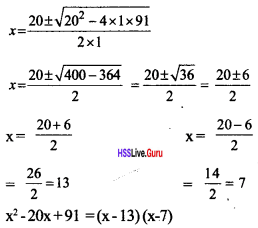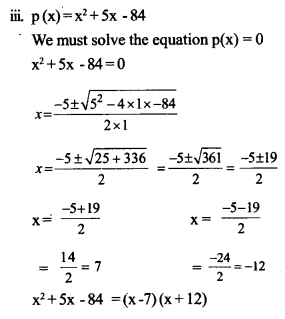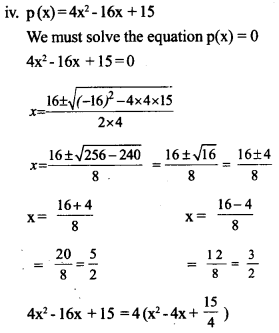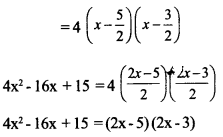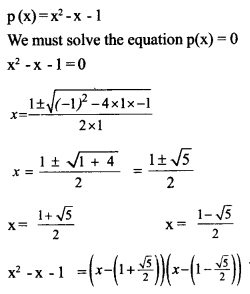Polynomials Class 10 Question 2. Prove that none of the polynomials below can be factored into a product of first degree polynomials:
i. x2 + x + l
ii. x2 – x + l
iii. x2 + 2x + 2
iv. x2 + 4x + 5
i. x2 + x+1
We must solve the equation p(x) = 0
x2 + x +1 =0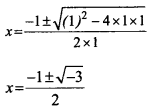no solutions
p(x) doesn’t have any first degree factors.
ii. x2 – x + 1
We must solve the equation p(x) = 0
x2 – x + 1 = 0no solutions
p(x) doesn’t have any first degree factors
iii. x2 + 2x + 2
We must solve the equation p(x) = 0
x2 + 2x + 2 = 0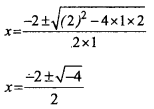no solutions
p(x) doesn’t have any first degree factors.
iv. x2 + 4x + 5
We must solve the equation p(x) = 0
x2 + 4x + 5 = 0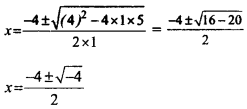no solutions
p(x) doesn’t have any first degree factors.

Maths Solutions Question 3. In the polynomial p(x) = x2 + 4x + k, up to what number can we take ask, so that p(x) can be factorized as a product of two first degree polynomials?
p(x) = x2 + 4x + k
p(x) = 0
x2 + 4x + k = 0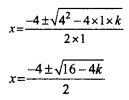p(x) can be factorized as a product of two first degree polynomials, the $$\sqrt{16-4 k}>0$$ So, k can take value up to 4.

### Polynomials Orukkam Questions & Answers

Worksheet 1

Kerala 2x Question 1. Write the product (x – 1) x (x + 1)
Find the product of (x – 1),(x + 1),(x + 2) If the poduct is p(x)find p(1), (-1), p(-2) Write the solution of the equation p(x) = 0.
(x – 1)x (x + 1) = x2 – 1
(x – 1) (x + 1) (x + 2)=(x2 – 1) (x + 2)
= x3 – x + 2x2 – 2 = x3 + 2x2 – x – 2 = 0
p(x) = x3 + 2x2 – x – 2 = 0
p(1) =1 + 2 – 1 –  2 = 0
p(–1) = – 1 + 2 + 1 – 2 = 0
p(–2) = – 8 + 8 + 2 – 2 = 0
1, – 1, – 2 are the solutions of the equation p(x) = 0.Question 2.
Expand (x – a)(x – b). If x2 – 7x + 12 = (x – a)(x – b) then find a+b.
Also find ab Calculate the values of a, b. Write the factors of (x2 – 7x +12 ).
Find the solutions of (x2 – 7x + 12).
(x – a) (x – b) = x2 – bx – ax + ab
= x2 – x (a + b) + ab
= x2 – 7x + 12 = (x – a)(x – b)
a + b = 7
ab = 12
(a + b)2 – 4ab = 72 – 4 x 12 = 49 – 48 = 1 = a – b
a + b = 7
a – b = 1, 2a = 8 a = $$\frac { 8 }{ 2 }$$ =4
b = 7 – 4 = 3 a = 4 and b = 3.
Solution of x2 – 7x + 12 is x2 – 7x + 12 = (x – 4) (x – 3)
Solutions = 4, 3

Question 3.
If p(x) = x3 – 6×2 + 11x – 1 then find p(1), p(2), p(3). Find p(x) – p(1), p(x) – p(2), p(x) – p(3), p(x) – p(1). Write the solutions of p(x) – p(1) = 0
Polynomials Class 10 Worksheet with Answer:
p(x) = x3 – 6x2 + 11x – 1
p(1) = 1 – 6 + 11 – 1 = 5
p(2) = 8 – 24 + 22 – 1 = 5
p(3) = 27 – 54 + 33 – 1 = 5
p(x) – p(1) = x3 – 6x2 + 11x – 6
p(x) – p(2) = x3 – 6x2 + 11x – 6
p(x) – p(3) = x3 – 6x2 + 11x – 6
p(x) – p(1) = x3 – 6x2 + 11x – 6 = 0
If x = 1, 2, 3 then p(x) – p(1) = 0.
Factors of the equations are (x – 1), (x – 2), (x – 3).
Solutions of the equations are 1, 2, 3.

Question 4.
When p(x)is divided by (ax + b), the quotient is q(x)and the remainder is c. p(x) = (ax + b) x q(x) + c
When does the value of p(x) equal to c $$p\left(\frac{-b}{a}\right)=\left(a \times \frac{-b}{a}+b\right) \times q\left(\frac{-b}{a}\right)+c$$
What is the remainder when p(x)is divided by ax + b. When does(ax + b)becomethe factor of p(x).
The remainder obtained when p(x) is divided by (ax + b) = $$p\left(\frac{-b}{a}\right)$$
When $$p\left(\frac{-b}{a}\right)$$ = 0, then (ax + b) will be a factor of p(x).

Worksheet 2

Question 5.
Write the following as the product of first degree polynomials
1. x2 + 7x + 12
2. x2 + 3x + 2
3. x2 – 9x – 22
4. 2x2 + 5x – 3
1. x2 + 7x + 12 = (x + 4)(x + 3)
2. x2 + 3x + 2 = (x + 2)(x + 1)
3. x2 – 9x – 22 =(x – 11)(x + 2)
4.2x2 + 5x – 3 =$$\left(x-\frac{1}{2}\right)$$(x+3)=(2x-l)(x+3)

Question 6.
Write a polynomial p(x) in which p(1) = 0, P(-2) = 0, p(2) = 0.
If p( 1) = 0, then x – 1 is a factor.
If p(–2) = 0, then x + 2 is a factor.
If p(2) = 0, then x – 2 is a factor.
p(x) = (x – 1) (x + 2) (x – 2) = x3 – x2 – 4x + 4

Question 7.
Write a second degree polynomial p(x) in which $$p(\sqrt{2}+1)=p(\sqrt{2}-1)=0$$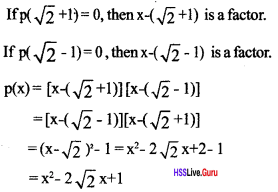Question 8.
Prove that x2 + 2x +2 cannot be written as the product of first degree polynomials
b2 – 4ac = 22 – 4 x 1 x 2 = –4 < 0
∴ x2 + 2x + 1 cannot be written as the product of first degree polynomials

Worksheet 3

Question 9.
Find the remainder and quotient obtained by dividing x3 – 5x2 + 7x + 3by (x + 2).
Let quotient = x2 + ax + b and remainder be c, then
x3 – 5x2 + 7x + 3 =(x + 2)(x2 + ax + b) + c +
= x3 – 5x2 + 7x + 3 = x3 + ax2 + bx + 2x2 + 2ax + 2b + c
= x3 +( a + 2) x2 + (b + 2a)x + 2b + c From this equation,
a + 2 = –5,
b + 2a = 7, 2b + c = 3
i.e., a = –7
b= 21
c = -39
quotient = x2 – 7x + 21,
remainder = –39Question 10.
Given x – 1 is a factor of x2 + ax + b. Prove that (a + b = –1)
Let (x – 1) be a factor, then p(1)=0
p(1) = 12 + a x 1 + b = 0
i.e., a + b = –1

Question 11.
p(x) = (4x2 – 1)(x + 2)Write p(x) as the product of first degree factors.Write p(x) in the form of a trird degree polynomial What is the remainder obtained by dhpding 4x3 + 6x2 – x + 2by (x + 2) .What is the re¬mainder obtained by dividing 4x3 + 6x2 – x + 1 by (2x – 1).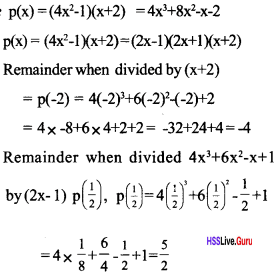### Polynomials SCERT Questions & Answers

Question 12.
Write the second-degree polynomial p(x)= x2 + x – 6 as the product of first-degree polynomials. Find also the solution of the equation p (x)=0 [Score: 4, Time: 7 minute]Question 13.
For what values of x, the polynomial 2x2 – 7x – 15 is equal to zero? Write this polynomial as the product of two first degree polynomials. [Score: 4, Time: 7 minute]Question 14.
Write the polynomial p(x) = x2 + 4x + 1 as the product of two first degree polynomials. Find the solution of the equation p (x) = 0. [Score: 4, Time: 5 minute]
x2 + 4x + 1 = (x – a) (x – b) = x2 – (a + b) x + ab(1)
a + b = –4, ab = 1, a – b = 2√3
a = –2 + √3, b = –2 – √3 (1)
x2 + 4x + 1 =(x + 2+ √3 ) (x + 2 – √3 ) (1)
x2 + 4x + 1 = 0 =>(x + 2 + √3 )(x + 2 –√3 ) = 0 (1)
x = –2 – √3 ,or x = –2 + √3

Question 15.
In the polynomial p (x) = x2+ ax + b p (3 + √2 )= 0, p (3 – √2 ) = 0, write this polynomial after finding a and b. [ Score: 4, Time: 5 minute]
p (x) = x2 + ax + b
p(3 + √2) = 0, (x – 3 – √2) is a factor (1)
p(3 – √2 ) = 0, ( x – 3 + √2) is a factor (1)
p(x) = x2 + ax + b = (x – 3 – √2) (x – 3 + √2)
= (x – 3)2 – ( √2)2 (1)
x2 + ax + b = x2 – 6x + 7 (1)

Question 16.
What number should be added to the polynomial p(x) = x2 + x – 1, so that (x – 2)is a factor of the new polynomial. [Score: 4, Time: 6 minute]
p (x) = x2 + x – 1, remainder p(2) (1)
p (2) = (2)2 + 2 – 1 = 5 (1)
For x – 2 to become a factor of p (2) must be equal to zero.
For p (2) = 0 here we have to substract 5 ffomp(x). (1)
That is, add –5 to p(x) for (x – 2) become a factor. (1)

Question 17.
What is the smallest natural number k, for which the polynomial 2x2 + kx + 6 can be written as a product of two first degree polynomials? Write down the polynomial using k and express it as the product of two first degree polynomials [Score: 4, Time: 8 minutes]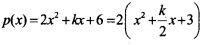Question 18.
Method to check whether(x – a), and (x + a)are factors of a polynomial P(x).
Check whether (x + 2) and (x – 5) are factors of the polynomial p(x) = x2 + 7x + 10 [Score: 4, Time: 6 minute]
When a polynomial p(x) is divided by (x – a), if p(a) = 0 then (x – a) is a factor of p(x). When a polynomial p(x) is divided by (x + a), if p(–a) = 0 then (x + a) is a factor of p(x).
p(x) = x2 + 7x + 10
p(–2) = 4 – 14 + 10 = 0 (1)
∴ x + 2 is a factor (1)
Remainder p(5) = (5)2 + 7(5) +10 (1)
= 25 + 35 + 10 ≠ 0 (1)
∴ x – 5 is not a factor

Question 19.
When dividing x2 + ax + b by (x – 2) and (x – 3) the remainder is zero. What are the numbers a and b. [Score: 3, Time: 5 minute]
p(x) = x2 + ax + b = (x – 3)(x – 2)
= x2 – 5x + 6
a = –5, b = 6 (3)

### Polynomials Exam Oriented Questions & Answers

Short Answer Type Questions (Score 2)

Question 20.
Check whether x – 1 is a factor of 3x3 – 2x2 – 3x + 2.
P(1)=3 x 13 – 2 x 12 – 3 x 1 + 2 = 0
Therefore x – 1 is a factor.

Question 21.
If (x – 1) is to be a factor of p(x) = a2x2 – 4ax + 4a – 1. What should be the value of ‘a’ ?
p(1) = 0
a2 – 4a + 4a – 1 = 0
a2 – 1 =0
a = +1 or –1

Question 22.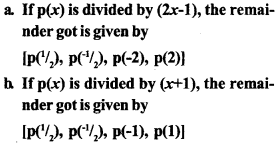Question 23.
Prove that (x – 1) is a factor of x13 – 1
p(x) = x13 – 1
p(1)= 113 – 1 = 1 – 1=0
p(1) = 0
(x – 1) is a factor of p(x)

Question 24.
Write the solution of polynomials p(x) = x2 – 7x + 12.
x2 – 7x + 12 = (x – 4)(x – 3)
p(x) = 0 (x – 4) ( x – 3) = 0
x = 4, x = 3

Question 25.
The quotient is x2 – 5x + 6 when the polynomial p(x) is divided by (x – 1), and the remainder is 7. Then
a. p(x) = (………. ) ( ……… ) + 7. Complete it
b. Find P(2).
a. p(x) = (x2 – 5x + 6) (x – 1) + 7
b. p(2) = 4 – 10 + 6 + 7 = 7

Short Answer Type Questions (Score 3)

Question 26.
a. Find the remainder when
x3 – 4x3 + 12x – 45 is divided by (x – 2)?
b. Find the value of k if the remainder is zero on dividing 2x3 + 4x2 – 10x + k by (x – 1)
a. p(x) = x3 – 4x2 – 12x – 45
remainder = p(2) = 23 – 4(2)2 + 12 x 2 – 45 = 32 – 61 = –29
b. p(x) = 2x3 + 4x2 – 10x + k; p(l) = 0
=> 2 x 13 + 4 x 12 – 10 x 1 + k = 0
2 + 4 – 10 + k = 0 – 4 + k = 0; k = 4Question 27.
Factorise 3x2 + 5x + 2 completely.Question 28.
Which first-degree polynomial is added to the polynomial 5x3 + 3x2 to get x2 – 1 as a factor.
p(x) = 5x3 +3x2 + ax + b
∴ x2 – 1 isafoctorthen p(1), p(–1) will be zero.
p(1) = 5 x 1 + 3 x 1 + a x 1 + b = 0
= 5x – 1 + 3 x 1 + ax – 1 + b = 0
a + b = –8 (1)
= 5x – 1 + 3 x 1 + ax – 1 + b = 0
–a + b = 2 (2)
Find the solutions of the equation
b = –3, a = –5 added polynomial = –5x – 3

Long Answer Type Questions (Score 4)

Question 29.
a. Find the value of k if remainder when 5x3 + 4x – 11x + k is divided by (x – 1) is 0.
b. When x3 – 2x2 + kx + 7 is divided by (x – 4) remainder is 11. Find k.Question 30.
a Show that the polynomial x + x + 1 has no first degree factors,
b. What is the remainder when the polynomial (x – 1) (x – 2) (x – 3) is divided by (x – 1)?
c. When (x – 1) (x – 2) (x – 3) + 2x + k is divided by (x – 1), the remainder is 10.
Then find out the remainder when it is divided by (x – 2).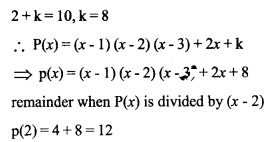Long Answer Type Questions (Score 5)

Question 31.
Write 2x2 + 5x + 3 as a product of two first degree polynomials.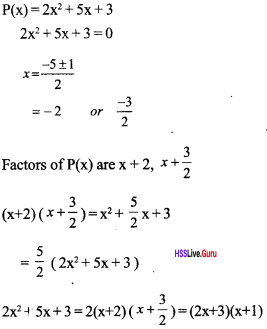.